In our last article, we defined the physics basics of a car negotiating a corner at constant speed and introduced a simplified vehicle model to explain the forces exchanged with the road. Before we move a step forward with our simplified vehicle model, we have to introduce some key players in this game.The picture above shows how the velocity vector V has two components, in car’s coordinate system: a longitudinal one, u and a lateral one, v. Body slip angle β depends on v and u: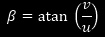β is normally pretty small in circuit racing so that we can assume it is simply the ratio between u and v; moreover, if we accept the small angles approximation, we also have that, in most cases: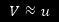We’ll now move a step forward and consider our bicycle model moving on a circular path with a radius R, but with a higher (although still constant) speed V (or u), so that tyres slip angles cannot be neglected anymore.

Our simplified vehicle finds itself in what engineers call “steady state”, which means, in cornering, moving on a perfectly circular path of fixed radius, at constant forward speed and with the only lateral acceleration acting on the car. Consequently, the net Yaw Moment acting on the car will be equal to zero, as we have seen in our previous entry.

We will also assume that the front and rear tyres are exactly the same and that the CG seats in the middle of the wheelbase, or, in other terms, at the same distance to the front and rear axle. This means, our vehicle has a perfectly balanced weight distribution, with half of the static weight acting on the front axle and the other half on the rear.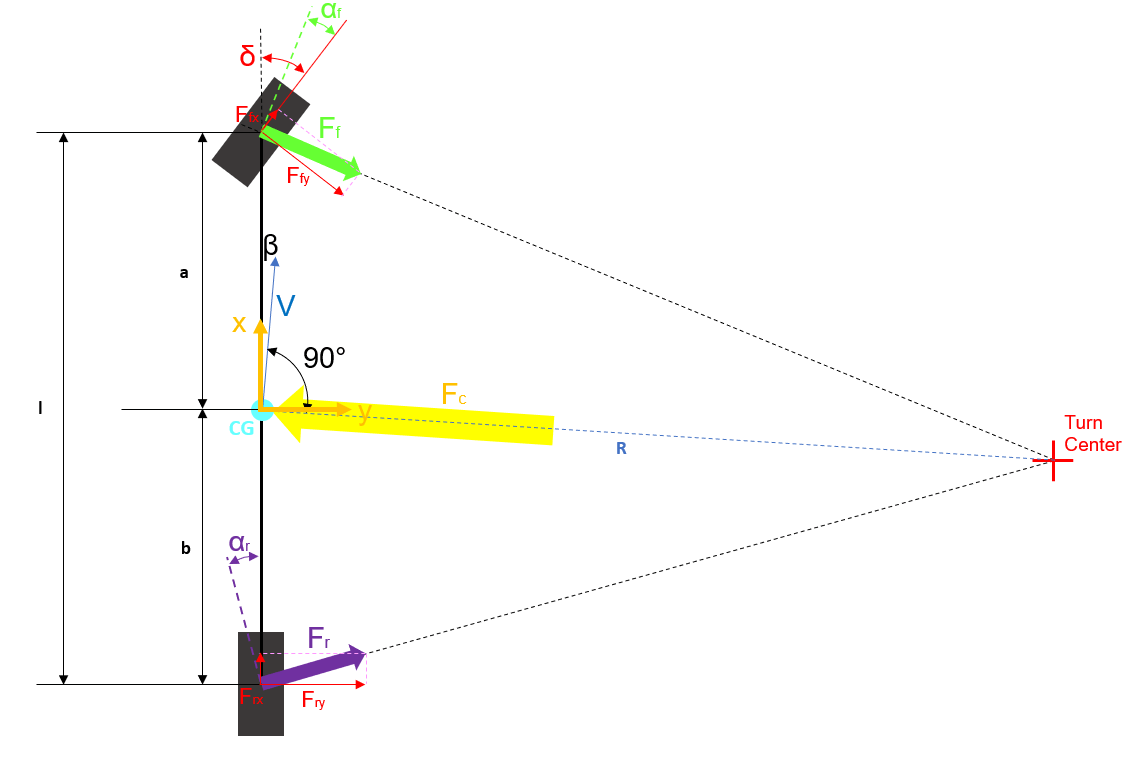As the previous image shows, since the car has exactly half of the weight acting on each wheel (we ignore here any aerodynamic action) and the tyres are identical, we can conclude that the forces developed by each tyre will also be the same (otherwise there would be no equilibrium) and the sum of their magnitudes must be equal to that of the centrifugal force Fc acting at the CG. In particular, for this to be true, we have to assume that the small angle approximation is valid, which means we will work considering all the angles being so small that the lateral component of each vector has more or less the same magnitude of the vector itself. Indeed, since racecars use often pretty small steering angles, this approximation is not a big error source but helps to simplify the analysis significantly. Under this assumption, to have both force and moments equilibrium, both front and rear forces must have the same magnitude because “a” and “b” have the same length.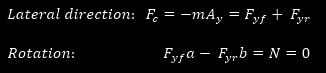To be absolutely correct, we should consider also the equilibrium in the longitudinal direction. However, this is not particularly important now.

According to the two equations above, we can also conclude that front and rear slip angles are the same, because, given a fixed vertical load, for the tyres to produce the same cornering force, the same slip angle would also be required. Therefore, to travel on a circular path of radius R, our idealized vehicle model will always require a steering angle δ equal to δack, independently from velocity V.

To understand why, we can imagine the complete vehicle being rotated anticlockwise by an angle equal to rear slip angle αr (and, hence, equal also to the front slip angle) with respect to the condition it had at very low forward velocity (previous article): this is the reason why in the above picture the corner center moves forward with regards to car local coordinate system and compared to where it was for the vehicle cornering at very low speed.

This rotation is also what steers the front wheels by the front slip angle, again here equal to the rear one.A vehicle behaving this way is normally described as neutral because the steering angle required to negotiate a corner always stays the same and remains equal to the Ackermann angle. To be exact, this definition is actually valid only if the tyres work in their linear range, which means in a point of the curve cornering force vs slip angle where the curve itself has not bent downward yet.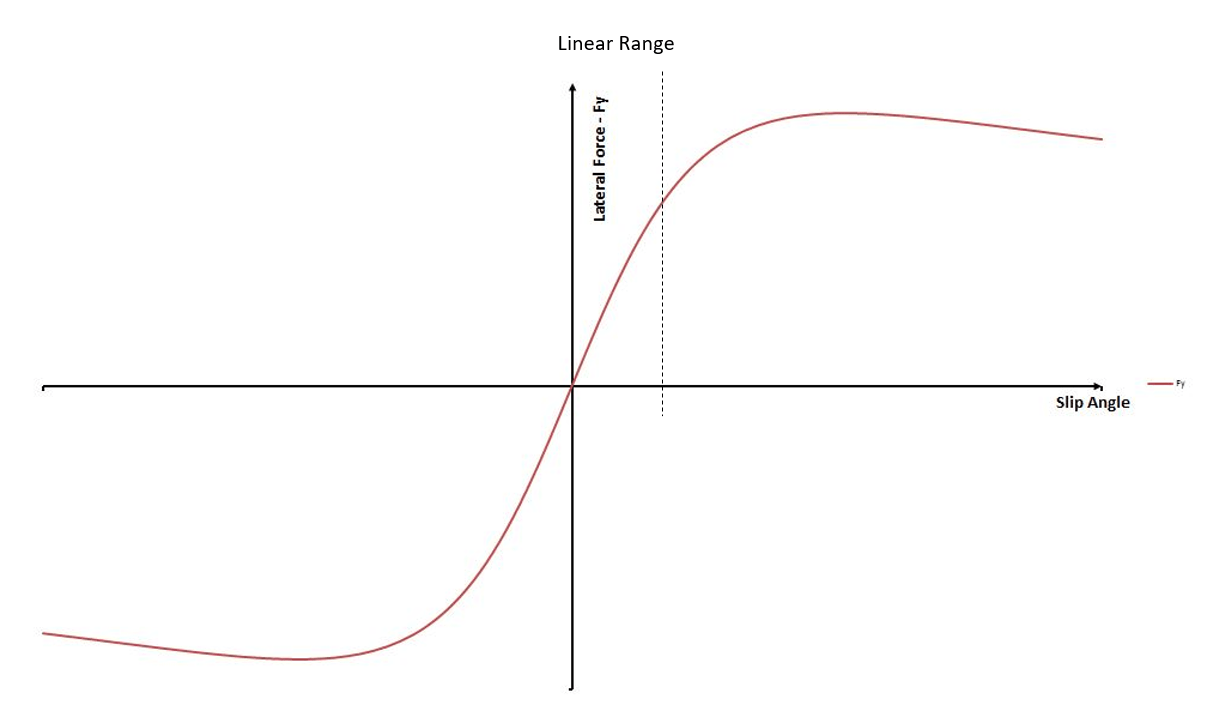Assuming the tyres are working in their linear range, we can rewrite our equilibrium equation in the lateral direction as: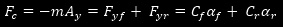This formulation emphasizes the importance of tyres cornering stiffness (see our previous entry for cornering stiffness definition) on cars handling and behaviour. For a car with tyres working in their linear range, we can also define neutral steering as a condition where the gradient of the front and rear slip angle with respect to lateral acceleration, required to create equilibrium, is the same for both axles.The reason behind this is that, for a certain increase in car’s lateral acceleration, both front and rear tyres will have to produce the same increase in cornering force, in order to balance the centrifugal force acting at car’s CG. If the tyres work in the linear range, for this to be true, they will have to experience the same change in slip angle.

Actually, most often a racecar operates (or should operate) close to the edge of tyres performance, where this approach loses some of its meaning: the tyres develop their maximum grip in such a situation, but lose pretty much all their power to “control” the car, following driver inputs, like steering. We can visualize this by looking at the tyre slip curve, in the following picture. We can imagine how, anytime a driver alters the steering angle, he tries to move on a different slip angle value and, hence, on a different point of the curve. How much difference in terms of cornering force he will get, by changing the steering angle by a certain amount, will depend on where we are on the curve: if we are close to the origin, any small slip angle change will produce a big change in cornering force, because the slope of the curve is pretty big. On the other hand, if we are on the top part of the curve, where it reaches its maximum and then start to decrease, any fixed change in the slip angle produce a very small change in the cornering force: this means that the driver will have much less power in controlling what the car does by using the steering wheel.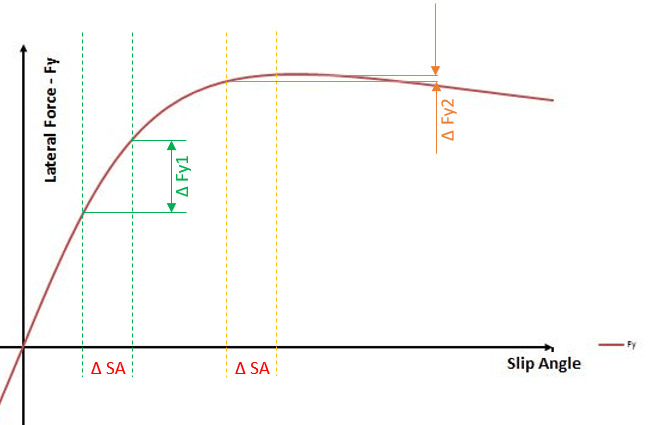This image shows how, for a given ΔSA (which is a variation of the Slip Angle), we obtain a much bigger ΔFy if we consider the green lines than if we consider the orange ones. This should show how, in the initial part of the curve (linear range), a fixed change in the slip angle produces much bigger effects on the cornering force than if we are closer to the edge of tyres performance (for a given vertical load). A detailed analysis of how a car behaves when closed to the tyres limit in terms of controllability and stability is, anyway, beyond the scope of this article. It is anyway an extremely important field in vehicle dynamics, above all when analyzing racecars, as they operated very often in this area of their performance envelope. The reader can get a feeling about this, when watching certain slow-motion videos where can be clearly recognized how the car more or less slides on the track: in similar situations, car’s path is normally decided by what the driver has done at the beginning of the cornering maneuver and is harder to influence once the car has been already pointed on a certain direction. With a neutral car, in particular, the driver will have in such a situation both front and rear axles at the limit of adhesion and this reduces dramatically what he can still do to control the car.

Nonetheless, this simple definition of a neutral vehicle can help to address some of the most used terms to characterize a racecar behaviour, sometimes also analyzing cars working close to their performance limit.

One important point that we mentioned already in our previous entry, analyzing a simplified car cornering at very low speed, is how the front tyre force points backward if we analyze it in a wheel or car local coordinate system. In our simple analysis we ignored the effects of this component, but its existence actually tells us how, for the car to effectively travel at constant speed, there must be a traction force (acting on the rear tyres, for a rear wheel drive car) pushing the vehicle forward and fighting (also) against the resistance created by the front (steered) tyres. This of course still ignores other forces like the rolling resistance (that we explained in our tyres series). This is the reason why, a car travelling through a very fast corner where high centripetal accelerations are achieved slows down a bit, compared if it would travel on a straight line: cornering generates resistive forces that play against the engine pushing the car forward.

Another interesting fact that can be noticed, analyzing our neutral simplified vehicle, is that the rear tyre moves on a circular path with a smaller radius than the one of the front tyre. This is unfortunately not too easy to detect, as the path of each wheel could not be drawn because too big for the picture. Anyway, a careful analysis of the image will show how the line connecting front wheel centre to turn centre (front wheel centre path radius) is slightly longer than the one connecting the rear wheel centre to the turn centre (rear wheel centre path radius).